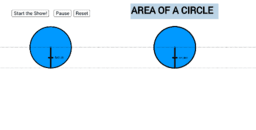# Area and Circumference Explained

Circumference and area formulas are explored to provide an informal argument for their formulas. Diameter is related to circumference, then radius is related to circumference. Then area of parallelogram and area of a triangle are explored to provide an informal argument for the circle area formula. Transformation of sections of a circle are shown to demonstrate the formula.
•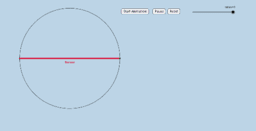### Circumference = How Many Diameters?

•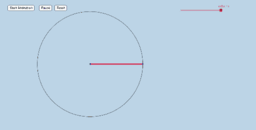### Circumference = How many Radii?

•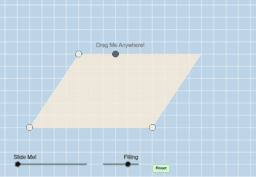### Parallelogram: Area

•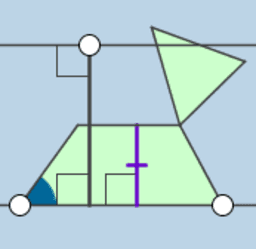### Triangle: Area

•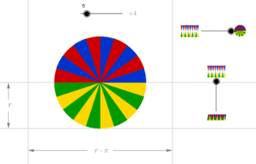### Area of a circle

•